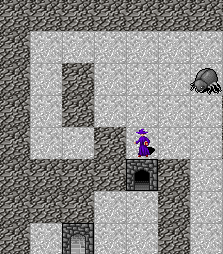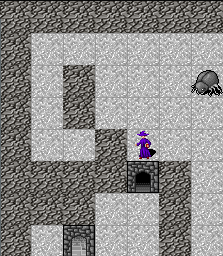Posted on April 2, 2018

# Something wrong

If you move the player over an open door, you may notice that something is not quite right.When the player is at the same position as the door, the door disappears. Whats interesting is that this does not happen to the background tiles.

Looking at the tile for the player, you can see that it has a transparent background. The UI drawing logic first draws the background tiles and then draws whatever tile we tell it to on top of that. When the player is on a blank tile you then get the player drawn with the background showing through. But when the player is on the door, then the current drawing logic replaces the door entity with the actor entity and so only the player’s tile is show.

However it is clear that the UI supports layering of images, so lets make use of this fact.

# Layers

Rather than sending a single set of entities to draw, lets send an array of layers. So change drMapData from a `[(Int, Int, Int)]` to a `[[(Int, Int, Int)]]`. I.e. from an list of positions to an array (layers) of list of positions.

###### 08_layers/src/GameCore.hs (88 to 92)
``````data UiDrawCommand = UiDrawCommand
{ drCmd :: !Text
, drScreenWidth :: !Int
, drMapData :: ![[(Int, Int, Int)]]
} deriving (Generic)``````

Then the UI draws each layer in order, i.e. the first layer is at the bottom the last is the top. This is achieved by adding a loop over the layers list and the drawing each layer as we did before.

###### 08_layers/html/rogue.js (115 to 135)
``````      case "draw": {
config.drawId = Math.random();

const colWidth = cmd.screenWidth;
const ctx = document.getElementById("tilesCanvas").getContext("2d");

//Draw background image of blank tiles
ctx.drawImage( getCachedBlankCanvas(), 0, 0 );

R.forEach( layer => {
R.forEach( ([atX, atY, tid]) => {
const [tX, tY] = tileFromTileId( tid );

//function drawTile( ctx, img, twidth, theight, dx, dy, trow, tcol ){
drawTile( ctx, tilesMain, config.tileWidth, config.tileHeight, atX, atY, tX, tY );

}, layer )
}, cmd.mapData );

break;
}``````

## drawAndSend

drawAndSend is changed to return an array of layers. I.e. from

`` drMapData = mkDrawMapData <\$> Map.toList playerTiles``

to

`` drMapData = mkDrawMapData <<\$>> (Map.toList <\$> layers)``

`<<\$>>` is Protolude’s infix for `(fmap . fmap)`. So here each layer is converted to a list, and then mkDrawMapData is called for each `(PlayerPos, Tile)` in each list.

###### 08_layers/src/GameEngine.hs (211 to 223)
``````drawAndSend :: World -> IO ()
drawAndSend world = do
let layers = drawTilesForPlayer world (world ^. wdMap)

let cmd = Ae.encodeText UiDrawCommand { drCmd = "draw"
, drScreenWidth = world ^. wdPlayer ^. plScreenSize ^. _1
, drMapData = mkDrawMapData <<\$>> (Map.toList <\$> layers)
}
sendData (world ^. wdPlayer ^. plConn) cmd

where
mkDrawMapData :: (PlayerPos, Tile) -> (Int, Int, Int)
mkDrawMapData (PlayerPos (x, y), tile) = (x, y, tile ^. tlId)``````

## drawTileForPlayer

drawTilesForPlayer does the work of creating the layers

###### 08_layers/src/GameEngine.hs (262 to 303)
``````drawTilesForPlayer :: World -> Map WorldPos Entity -> [Map PlayerPos Tile]
drawTilesForPlayer world entityMap =
let
-- Entity base layer
entityLayer = mkLayer entityMap

-- Actor layer on top
actorMap = Map.fromList \$ (\a -> (a ^. acWorldPos, a ^. acEntity)) <\$> getAllActors world
visibleActorMap = Map.filterWithKey inView actorMap
actorLayer = mkLayer visibleActorMap
in
[entityLayer, actorLayer]

where
player = world ^. wdPlayer

-- Top left of player's grid
(WorldPos (topX, topY)) = player ^. plWorldTopLeft

-- Players screen/grid dimensions
(screenX, screenY) = player ^. plScreenSize

-- Bottom right corner
(bottomX, bottomY) = (topX + screenX, topY - screenY)

inView (WorldPos (x, y)) _ =
x >= topX && x < bottomX && y > bottomY && y <= topY

mkLayer :: Map WorldPos Entity -> Map PlayerPos Tile
mkLayer entities =
let
-- Filter out blank
noEmptyMap = Map.filter (\e -> e ^. enTile ^. tlName /= "blank") entities

-- Only get the entitys that are at positions on the player's screen
visibleEntitys = Map.filterWithKey inView noEmptyMap

-- Get the tile for each entity
tileMap = (^. enTile) <\$> visibleEntitys
in
-- Get it with player positions
Map.mapKeys (worldCoordToPlayer \$ player ^. plWorldTopLeft) tileMap``````

To fix the issues only two layers are required. The bottom layer has the entities, and the top layer has the actors. The code for getting entities remains pretty much the same as before. The code for the actors is changed to create a separate layer for them.

###### 08_layers/src/GameEngine.hs (265 to 274)
``````  let
-- Entity base layer
entityLayer = mkLayer entityMap

-- Actor layer on top
actorMap = Map.fromList \$ (\a -> (a ^. acWorldPos, a ^. acEntity)) <\$> getAllActors world
visibleActorMap = Map.filterWithKey inView actorMap
actorLayer = mkLayer visibleActorMap
in
[entityLayer, actorLayer]``````

And it works# Changes

## src/GameCore.hs

`diff -w -B -a -d -u -b --new-file 07_collisions/src/GameCore.hs 08_layers/src/GameCore.hs`
`+++ 08_layers/src/GameCore.hs`
`@@ -86,10 +84,11 @@`
`                    deriving (Generic)`
` `
` `
`+`
` data UiDrawCommand = UiDrawCommand`
`                      { drCmd :: !Text`
`                      , drScreenWidth :: !Int`
`+                     , drMapData :: ![[(Int, Int, Int)]]`
`                      } deriving (Generic)`
` `
` `

## html/rogue.js

`diff -w -B -a -d -u -b --new-file 07_collisions/src/GameEngine.hs 08_layers/src/GameEngine.hs`
`+++ 08_layers/src/GameEngine.hs`
`@@ -210,13 +207,14 @@`
`   pure (x, y)`
` `
` `
`+`
` drawAndSend :: World -> IO ()`
` drawAndSend world = do`
`+  let layers = drawTilesForPlayer world (world ^. wdMap) `
`   `
`   let cmd = Ae.encodeText UiDrawCommand { drCmd = "draw"`
`                                         , drScreenWidth = world ^. wdPlayer ^. plScreenSize ^. _1`
`+                                        , drMapData = mkDrawMapData <<\$>> (Map.toList <\$> layers)`
`                                         }`
`   sendData (world ^. wdPlayer ^. plConn) cmd`
` `
`@@ -259,9 +258,23 @@`
`    PlayerPos (worldX - worldTopX, -(worldY - worldTopY))`
` `
`   `
`+`
`+drawTilesForPlayer :: World -> Map WorldPos Entity -> [Map PlayerPos Tile]`
` drawTilesForPlayer world entityMap =`
`+`
`   let`
`+    -- Entity base layer`
`+    entityLayer = mkLayer entityMap`
`+`
`+    -- Actor layer on top`
`+    actorMap = Map.fromList \$ (\a -> (a ^. acWorldPos, a ^. acEntity)) <\$> getAllActors world`
`+    visibleActorMap = Map.filterWithKey inView actorMap`
`+    actorLayer = mkLayer visibleActorMap`
`+  in`
`+    [entityLayer, actorLayer]`
`+`
`+`
`+  where`
`     player = world ^. wdPlayer`
`     `
`     -- Top left of player's grid`
`@@ -273,22 +286,17 @@`
`     -- Bottom right corner`
`     (bottomX, bottomY) = (topX + screenX, topY - screenY) `
` `
`+    inView (WorldPos (x, y)) _ =`
`+      x >= topX && x < bottomX && y > bottomY && y <= topY`
` `
`+    mkLayer :: Map WorldPos Entity -> Map PlayerPos Tile`
`+    mkLayer entities =`
`+      let`
`+        -- Filter out blank`
`+        noEmptyMap = Map.filter (\e -> e ^. enTile ^. tlName /= "blank") entities `
` `
`     -- Only get the entitys that are at positions on the player's screen`
`+        visibleEntitys = Map.filterWithKey inView noEmptyMap`
` `
`     -- Get the tile for each entity`
`     tileMap = (^. enTile) <\$> visibleEntitys `
`@@ -296,10 +304,6 @@`
`   -- Get it with player positions`
`   Map.mapKeys (worldCoordToPlayer \$ player ^. plWorldTopLeft) tileMap`
` `
` `
` getAllActors :: World -> [Actor]`
` getAllActors world =`
`+++ 08_layers/html/rogue.js`
`@@ -120,12 +121,14 @@`
`         //Draw background image of blank tiles`
`         ctx.drawImage( getCachedBlankCanvas(), 0, 0 );`
` `
`+        R.forEach( layer => {`
`         R.forEach( ([atX, atY, tid]) => {`
`           const [tX, tY] = tileFromTileId( tid );`
`           `
`           //function drawTile( ctx, img, twidth, theight, dx, dy, trow, tcol ){`
`           drawTile( ctx, tilesMain, config.tileWidth, config.tileHeight, atX, atY, tX, tY );`
` `
`+          }, layer )`
`         }, cmd.mapData );`
` `
`         break;`